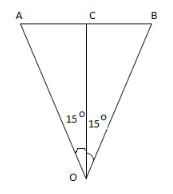# An aircraft is flying at a height of 3400m above the ground

An aircraft is flying at a height of 3400m above the ground. If the angle subtended at a ground observation point by the aircraft positions 10 s apart in 30o , what is the speed of the aircraft?The aircraft takes 10s to go from A to B and AB subtends an angle 30o at O.
In right angled triangle OAC,
AC = OC tan 15o = 3400 x 0.2679 = 911 m
Therefore, AB = 2 x 911 = 1822 m
Now, the speed of aircraft
v = AB/t = 1822 / 10 = 182.2 m/s